# Background

At first table calculations in Tableau are challenging to get your head around but are extremely useful. If you haven’t been exposed to them check out Andy Kriebel’s blog on setting them up. Due to their complex nature they often get a bad rep. I hope this blog, showing how to do table calculations in Alteryx vs Tableau, will help you appreciate Tableau’s table calculations more!

## The Challenge

We were tasked with the following task; in both Tableau and Alteryx calculate the percent of total cost of each age (1 to 100) within a data set. Take a look at the steps required for each software.

## The Alteryx Way

Figure 1 gives an overview of the workflow to calculate the percent of total cost for each age. This workflow started with inputting the data, a csv file. Next an autofield was used to convert numbers stored as strings to integers.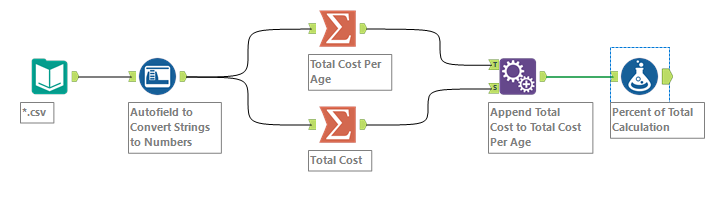Figure 1. The overall workflow to calculate the percent of total cost for each age.

After this the workflow needed to be split out and aggregated twice once to a per age level and once for an overall level. The summarise tool used to calculate the total cost per age is shown in Figure 2. The Data was grouped by age and the sum of cost for each age was taken. The total cost aggregation was achieved by summing only by cost in its respective summarise tool.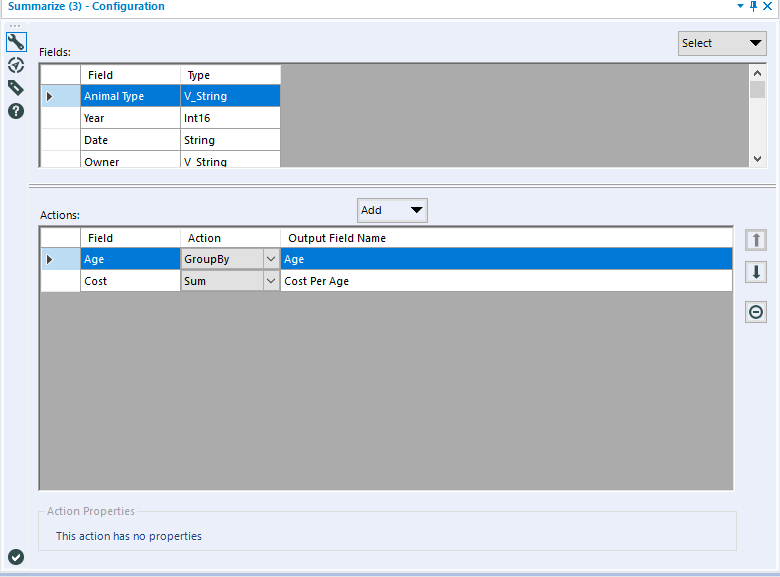Figure 2. Summarising cost data to each age.

The total cost was then appended to the cost per age. A simple calculation was used, dividing the cost per age by the total cost. This yielded the results in Figure 3, showing the percentage of total cost for each age.  Although you get to the answer this route is long winded the data is stored in your data source, not good. Now let’s look at how much easier it is in Tableau!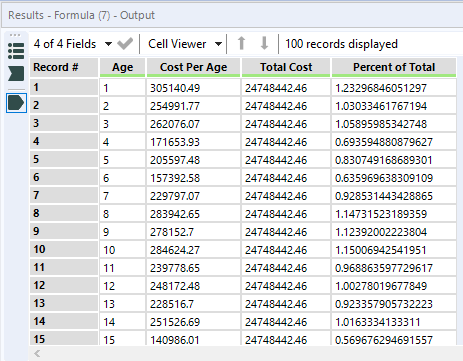Figure 3. The final output of the data.

# The Tableau Way

In Tableau simply put age and sum of cost into the view, as seen in Figure 4.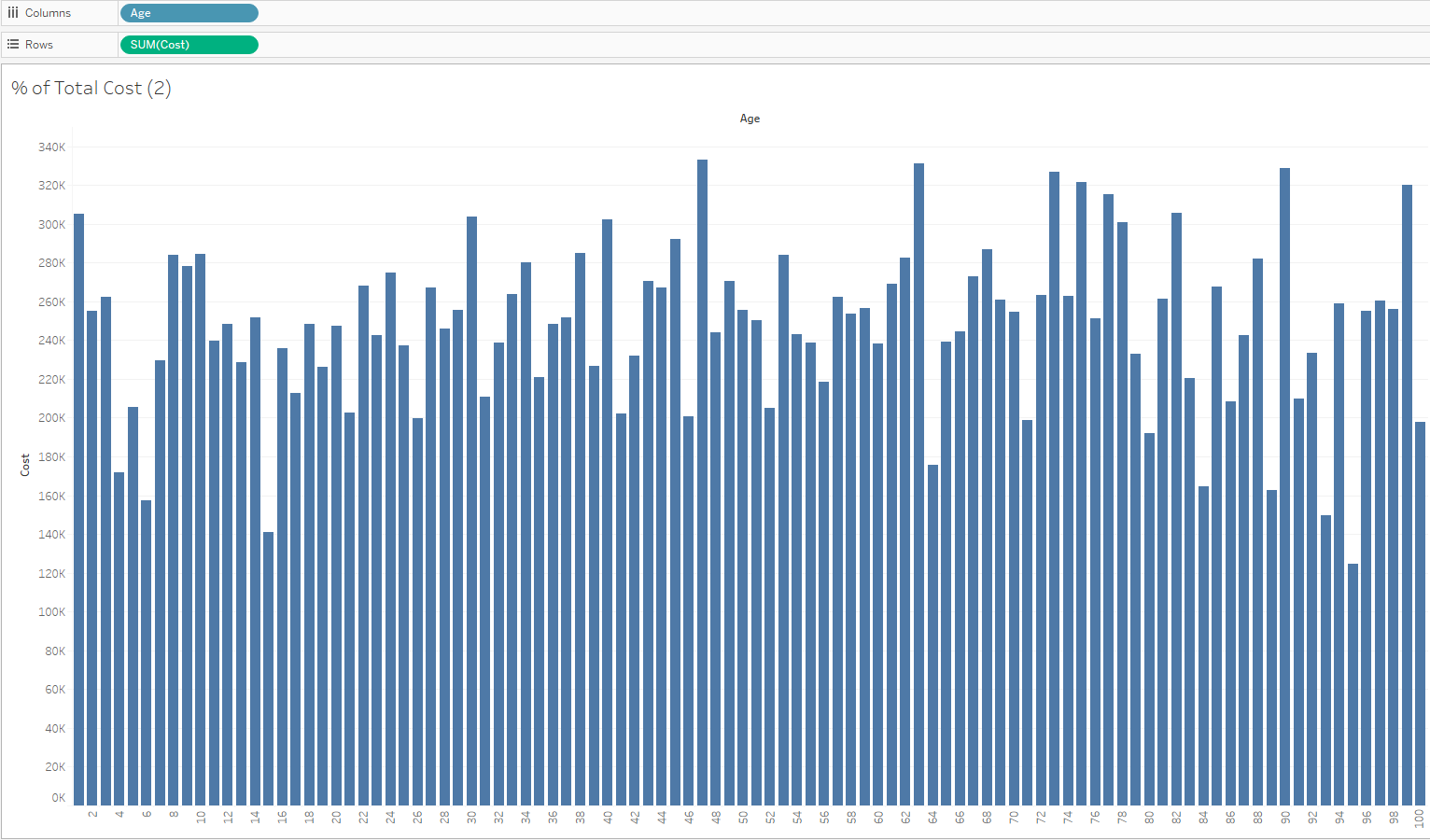Figure 4. The total cost for each age.

Next set up a table calculation by clicking the carrot on the age pill as shown in Figure 5. As there is only one dimension in the view select Quick Table Calculation and then Percent of Total.

.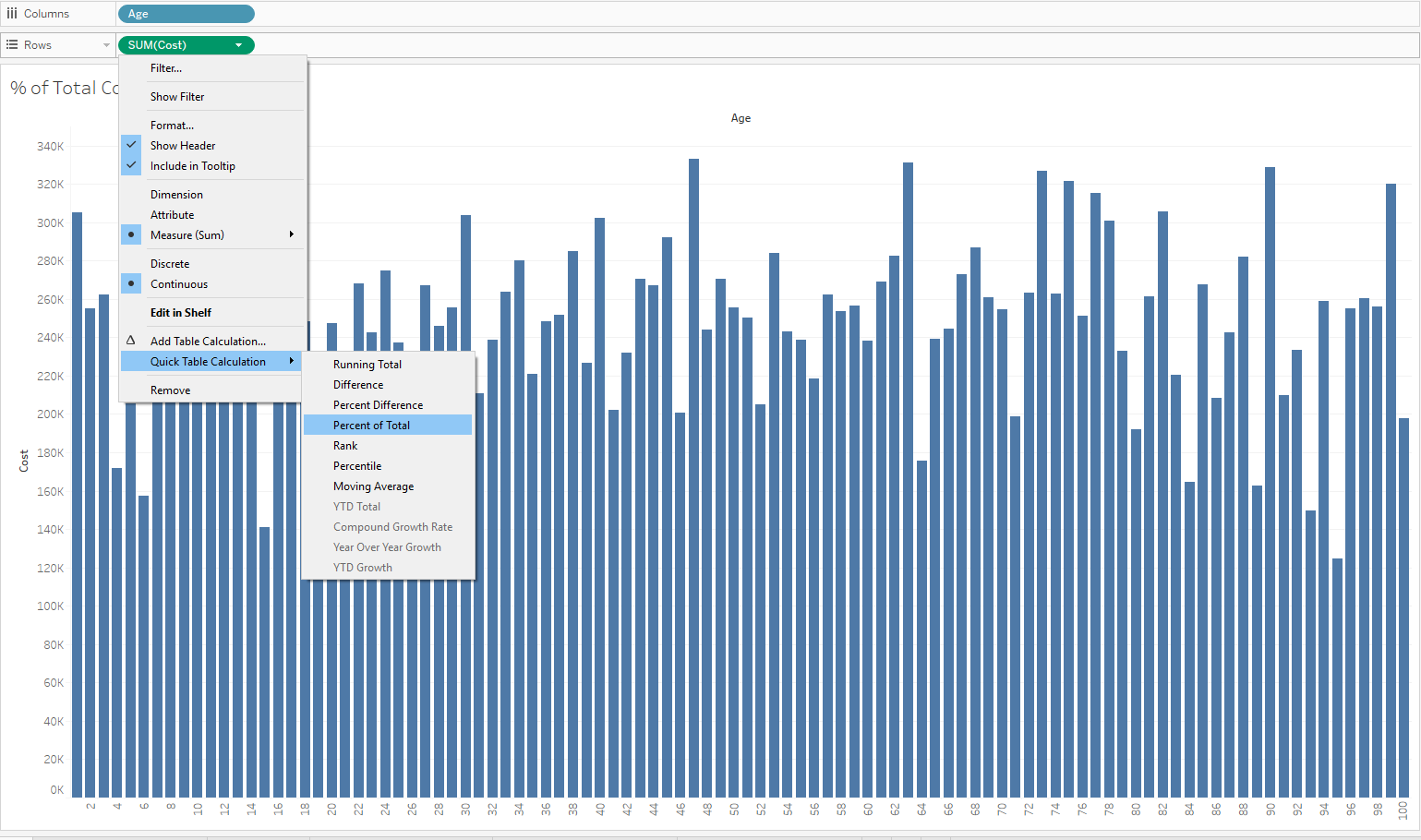Figure 5. Setting up the table calculation.

This produces the percent of total of each age in a lovely chart, as shown in Figure 6. Using Tableau is much quicker and easier than Alteryx. Bear this in mind next time you complain about making a table calculation!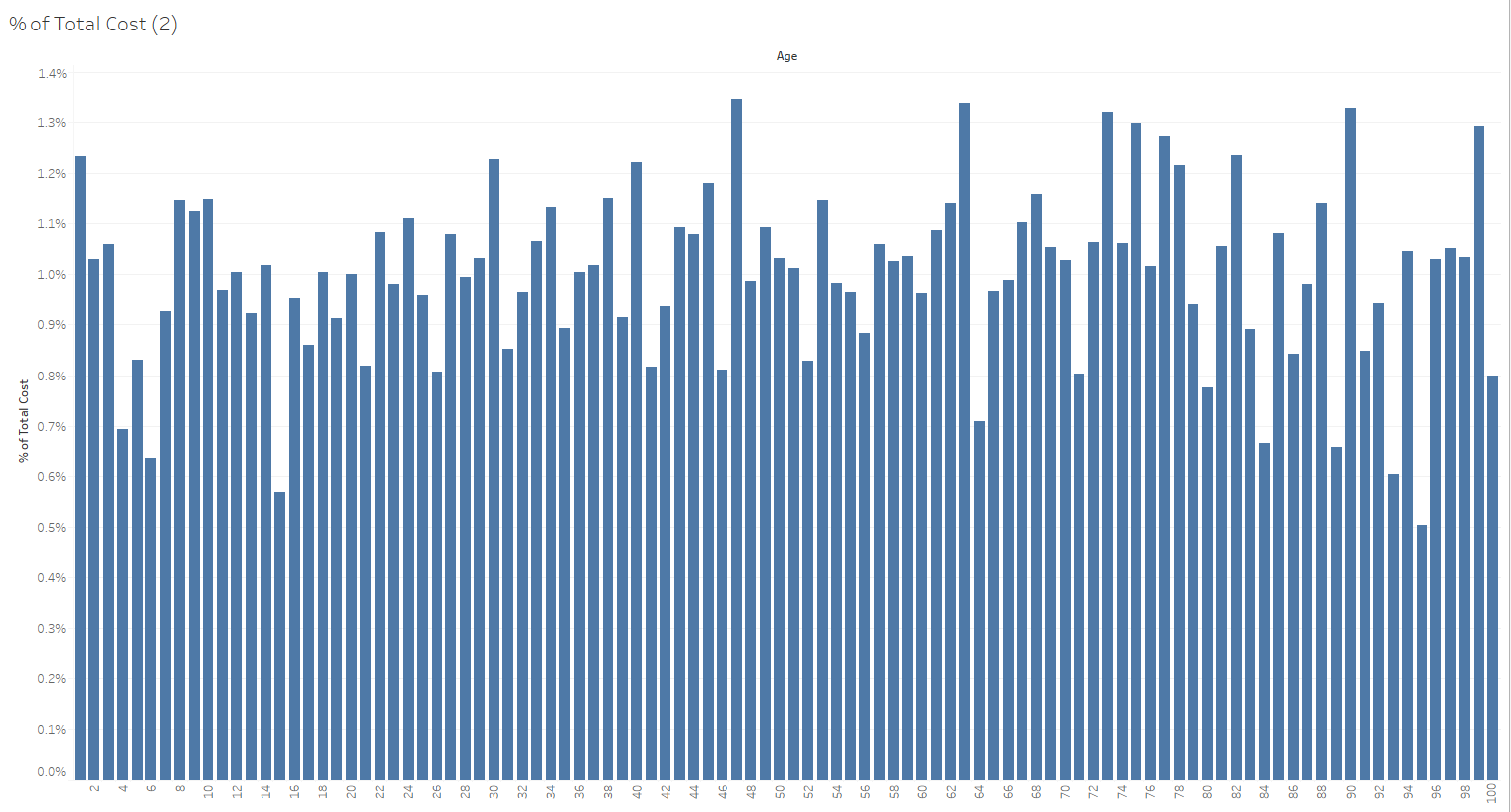Figure 6. Percent of Total of each age